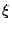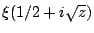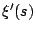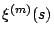# Statistics of the zeros of $\xi'(s)$

The Riemann-function is real on the 1/2-line and has all of its zeros there (assuming the Riemann Hypothesis). It is an entire function of order 1; because of its functional equation,is an entire function of order 1/2. It follows that the Riemann Hypothesis implies that all zeros ofare on the 1/2-line. (See [ MR 84g:10070] for proofs of these statements.) Assuming the Riemann Hypothesis to be true, one can ask about the vertical distribution of zeros of, and more generally of. It seems that the zeros of higher derivatives will become more and more regularly spaced; can these distributions be expressed in a simple way using random matrix theory? See the unpublished paper [Differentiation evens out zero spacings] of David Farmer.

Back to the main index for L-functions and Random Matrix Theory.